# Colleges with the highest SAT scores in Kansas

Top 10 colleges in Kansas with the highest SAT scores
Looking for the colleges with the highest SAT scores in Kansas? Well you're in luck! We've compiled a national college database and have created a list of the top 10 universities with the highest SAT scores in Kansas. These are the schools whose applicants had the highest average SAT scores in Kansas. And, since these tests are meant to determine academic prowess, they are arguably the schools with the most academically proficient students. You could even say these are the best colleges in Kansas. We also include each college's ACT scores and acceptance rate so that you can see where you would have the easiest or hardest time getting in. Read on to find out more.

## The University of Kansas SAT scores

The average SAT score for The University of Kansas is 1240.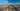The average SAT score of 1240 breaks down into:

• SAT math: 620

The average ACT score for The University of Kansas is 26 and their acceptance rate is 93.4%.

## Benedictine College SAT scores

The average SAT score for Benedictine College is 1170.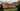The average SAT score of 1170 breaks down into:

• SAT math: 580

The average ACT score for Benedictine College is 25 and their acceptance rate is 92.8%.

## Kansas State University SAT scores

The average SAT score for Kansas State University is 1160.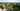The average SAT score of 1160 breaks down into:

• SAT math: 580

The average ACT score for Kansas State University is 24 and their acceptance rate is 94.6%.

## Newman University SAT scores

The average SAT score for Newman University is 1140.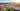The average SAT score of 1140 breaks down into:

• SAT math: 550

The average ACT score for Newman University is 23 and their acceptance rate is 63.8%.

## Wichita State University SAT scores

The average SAT score for Wichita State University is 1140.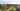The average SAT score of 1140 breaks down into:

• SAT math: 580

The average ACT score for Wichita State University is 23 and their acceptance rate is 97.1%.

## Pittsburg State University SAT scores

The average SAT score for Pittsburg State University is 1110.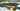The average SAT score of 1110 breaks down into:

• SAT math: 555

The average ACT score for Pittsburg State University is 22 and their acceptance rate is 87.2%.

## Washburn University SAT scores

The average SAT score for Washburn University is 1110.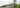The average SAT score of 1110 breaks down into:

• SAT math: 555

The average ACT score for Washburn University is 22 and their acceptance rate is 98.8%.

## McPherson College SAT scores

The average SAT score for McPherson College is 1090.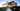The average SAT score of 1090 breaks down into:

• SAT math: 550

The average ACT score for McPherson College is 22 and their acceptance rate is 77.1%.

## Fort Hays State University SAT scores

The average SAT score for Fort Hays State University is 1080.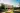The average SAT score of 1080 breaks down into:

• SAT math: 540

The average ACT score for Fort Hays State University is 21 and their acceptance rate is 89%.

## University of Saint Mary SAT scores

The average SAT score for University of Saint Mary is 1070.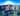The average SAT score of 1070 breaks down into:

• SAT math: 530